## RD Sharma Class 9 Solutions Chapter 17 Constructions MCQS

These Solutions are part of RD Sharma Class 9 Solutions. Here we have given RD Sharma Class 9 Solutions Chapter 17 Constructions MCQS

Other Exercises

Mark the correct alternative in each of the following:
Question 1.
The sides of a triangle are 16 cm, 30 cm, 34 cm. Its area is
(a) 225 cm²
(b) 225$$\sqrt { 3 }$$ cm²
(c) 225$$\sqrt { 2 }$$ cm²
(d) 240 cm²
Solution:
Sides of triangle and 16 cm, 30 cm, 34 cm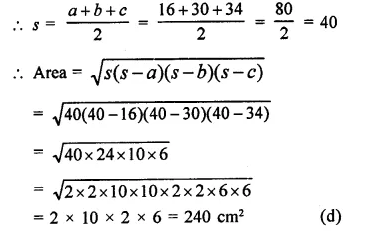Question 2.
The base of an isosceles right triangle is 30 cm. Its area is
(a) 225 cm²
(b) 225$$\sqrt { 3 }$$ cm²
(c) 225$$\sqrt { 2 }$$ cm²
(d) 450 cm²
Solution:
Base of isosceles triangle ∆ABC = 30cm
Let each of equal sides = x
Then AB = AC = x
Now in right ∆ABC,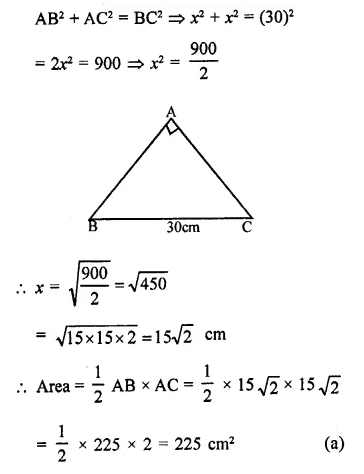Question 3.
The sides of a triangle are 7cm, 9cm and 14cm. Its area is
(a) 12$$\sqrt { 5 }$$ cm²
(b) 12$$\sqrt { 3 }$$ cm²
(c) 24 $$\sqrt { 5 }$$ cm²
(d) 63 cm²
Solution: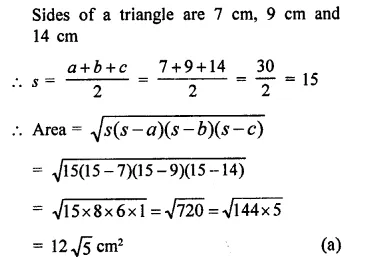Question 4.
The sides of a triangular field are 325 m, 300 m and 125 m. Its area is
(a) 18750 m²
(b) 37500 m²
(c) 97500 m²
(d) 48750 m²
Solution:
Sides of a triangular field are 325m, 300m, 125m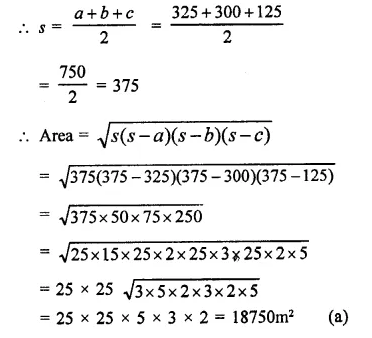Question 5.
The sides of a triangle are 50 cm, 78 cm and 112 cm. The smallest altitude is
(a) 20 cm
(b) 30 cm
(c) 40 cm
(d) 50 cm
Solution:
The sides of a triangle are 50 cm, 78 cm, 112cm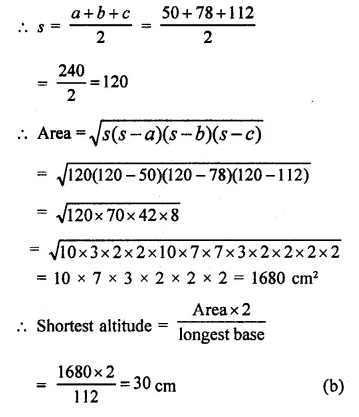Question 6.
The sides of a triangle are 11m, 60m and 61m. Altitude to the smallest side is
(a) 11m
(b) 66 m
(c) 50 m
(d) 60 m
Solution:
Sides of a triangle are 11m, 60m and 61m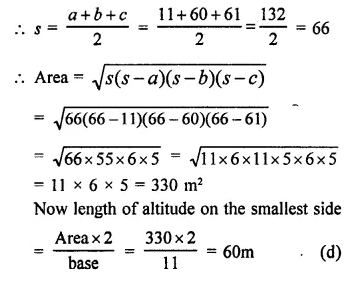Question 7.
The sides of a triangle are 11 cm, 15 cm and 16 cm. The altitude to the largest side isSolution:
Sides of a triangle are 11 cm, 15 cm, 16 cm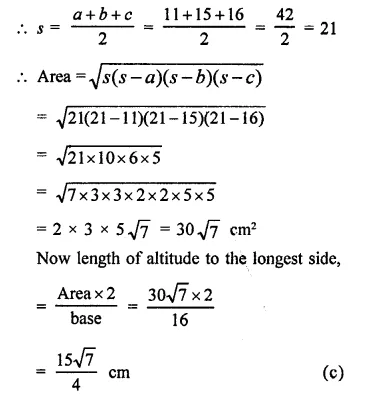Question 8.
The base and hypotenuse of a right triangle are respectively 5cm and 13cm long. Its area is
(a) 25 cm²
(b) 28 cm²
(c) 30 cm²
(d) 40 cm²
Solution:
In a right triangle base = 5 cm
base hypotenuse = 13 cm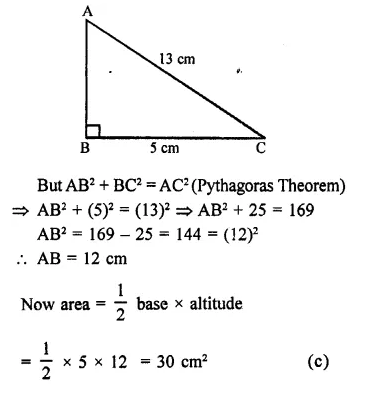Question 9.
The length of each side of an equilateral triangle of area 4 $$\sqrt { 3 }$$ cm², isSolution:
Area of an equilateral triangle = 4$$\sqrt { 3 }$$ cm²
Let each side be = a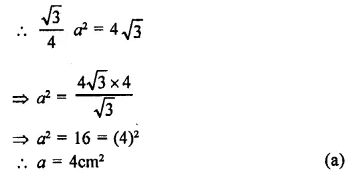Question 10.
If the area of an isosceles right triangle is 8cm, what is the perimeter of the triangle.
(a) 8 + $$\sqrt { 2 }$$ cm²
(b) 8 + 4$$\sqrt { 2 }$$ cm²
(c) 4 + 8$$\sqrt { 2 }$$ cm²
(b) 12$$\sqrt { 2 }$$ cm²
Solution:
Let base = x
ABC an isosceles right triangle, which has 2 sides same
⇒ Height = x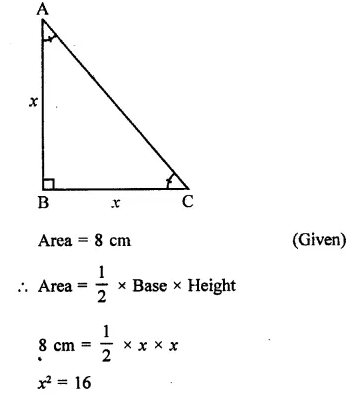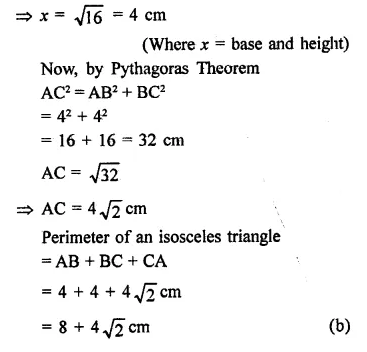Question 11.
The length of the sides of ∆ABC are consecutive integers. If ∆ABC has the same perimeter as an equilateral triangle with a side of length 9cm, what is the length of the shortest side of ∆ABC?
(a) 4
(b) 6
(c) 8
(d) 10
Solution:
Side of an equilateral triangle = 9 cm
Its perimeter = 3 x 9 = 27 cm
Now perimeter of ∆ABC = 27 cm
and let its sides be x, x + 1, x +2Question 12.
In the figure, the ratio of AD to DC is 3 to 2. If the area of ∆ABC is 40cm2, what is the area of ∆BDC?
(a) 16 cm²
(b) 24 cm²
(c) 30 cm²
(d) 36 cm²
Solution:
Ratio in AD : DC = 3:2
and area ∆ABC = 40 cm²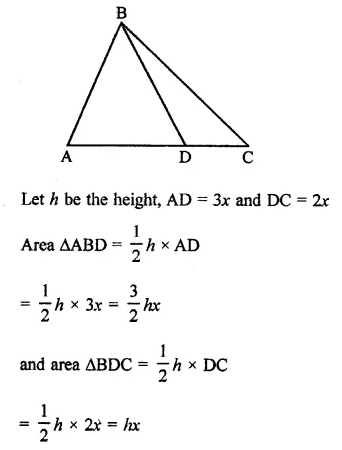Question 13.
If the length of a median of an equilateral triangle is x cm, then its area isSolution:
∵ The median of an equilateral triangle is the perpendicular to the base also,
∴ Let side of the triangle = aQuestion 14.
If every side of a triangle is doubled, then increase in the area of the triangle is
(a) 100$$\sqrt { 2 }$$ %
(b) 200%
(c) 300%
(d) 400%
Solution:
Let the sides of the original triangle be a, b, cQuestion 15.
A square and an equilateral triangle have equal perimeters. If the diagonal of the square is 1272 cm, then area of the triangle is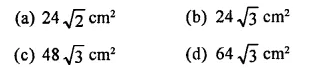Solution:
A square and an equilateral triangle have equal perimeterHope given RD Sharma Class 9 Solutions Chapter 17 Constructions MCQS are helpful to complete your math homework.

If you have any doubts, please comment below. Learn Insta try to provide online math tutoring for you.